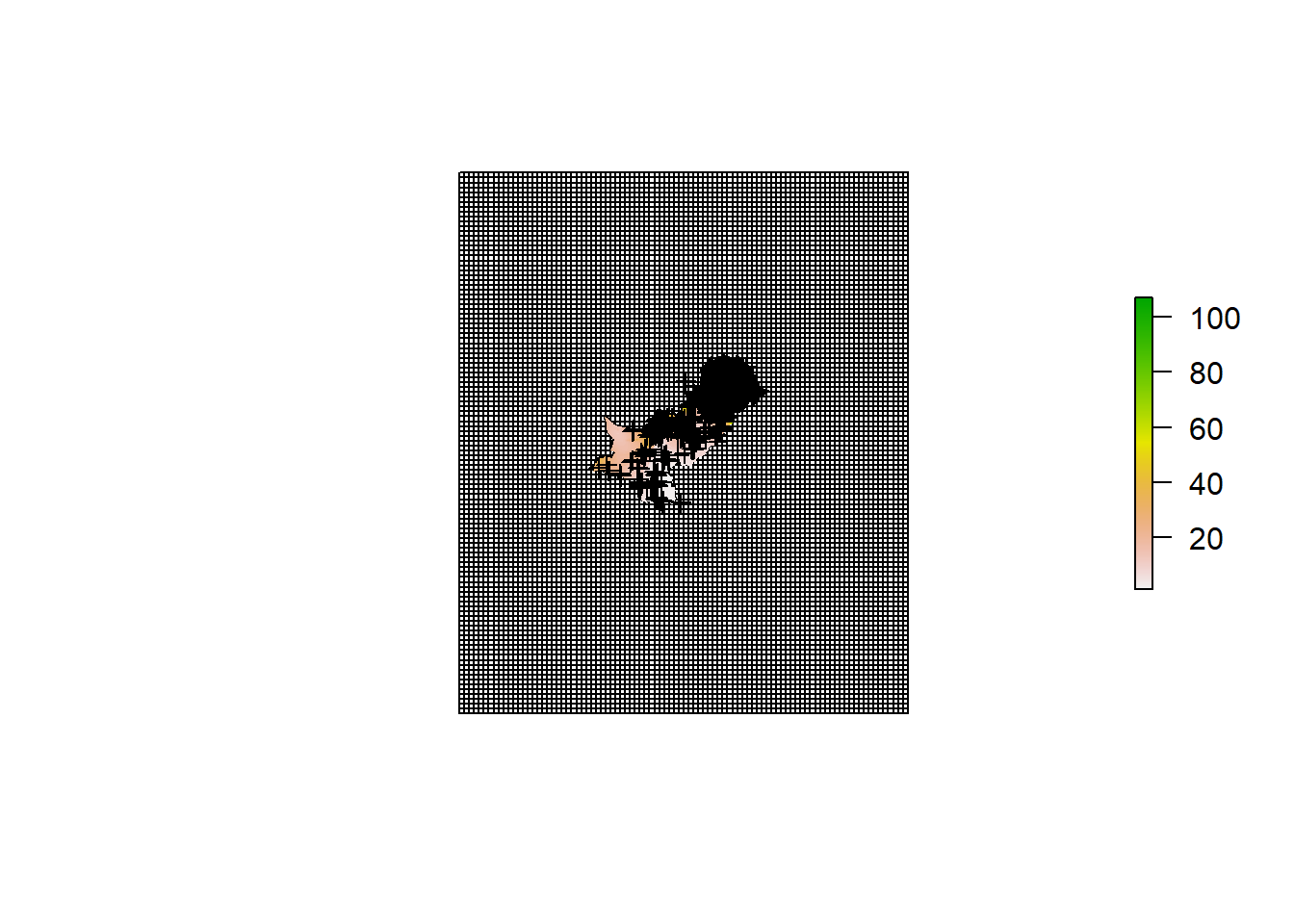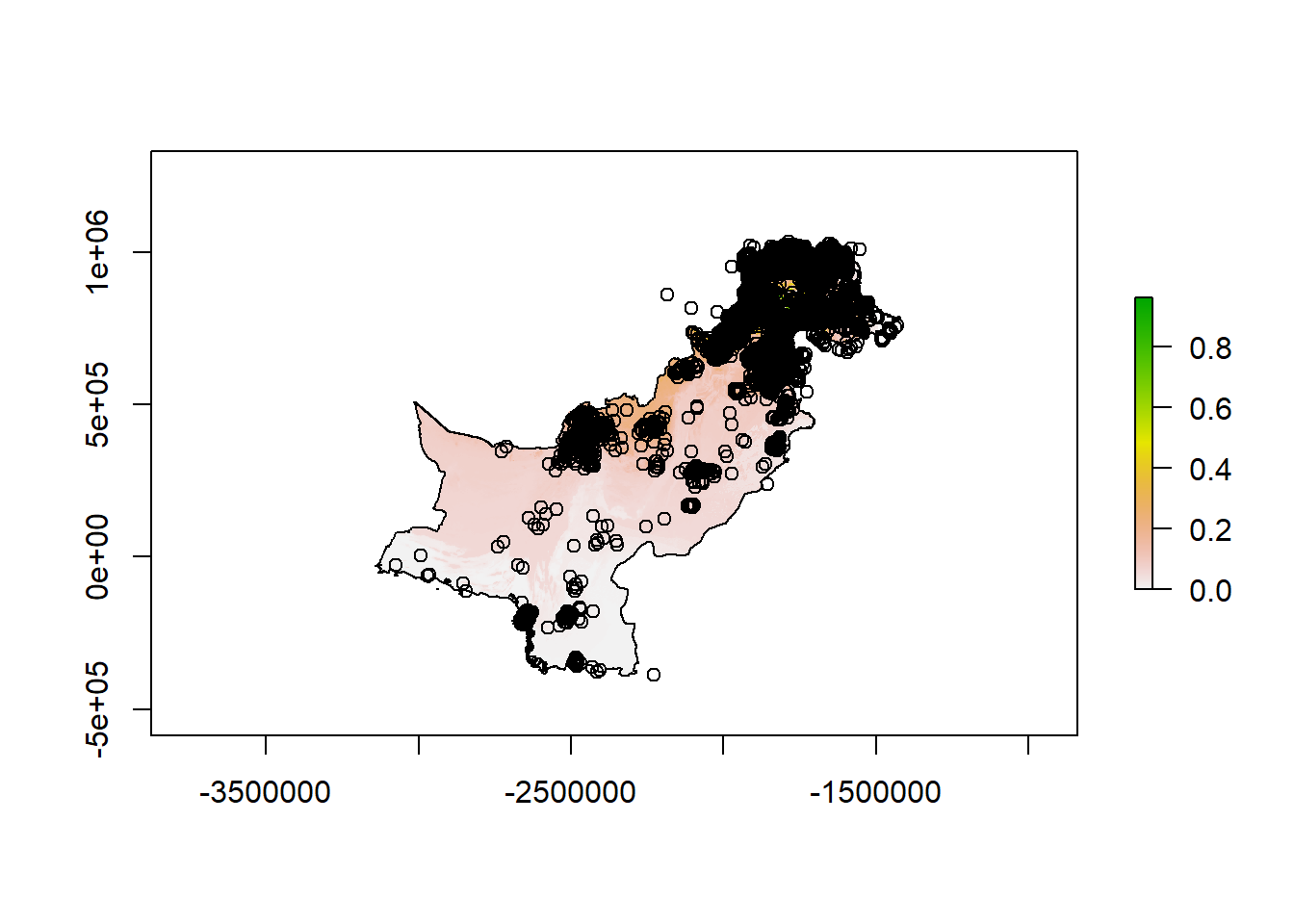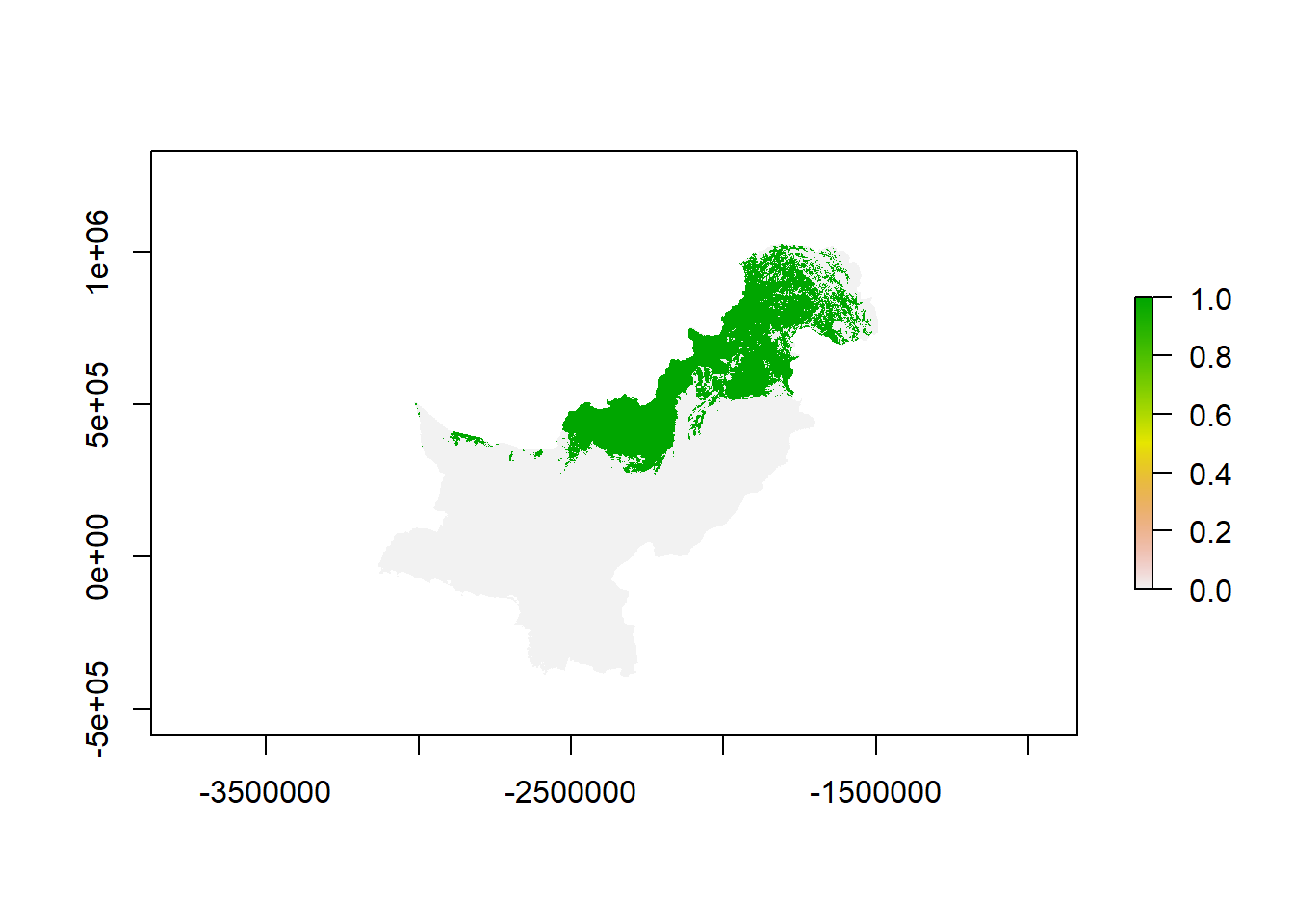# Distribution of Butterflies in PakistanAlt-Text

``library("sp")``
``## Warning: package 'sp' was built under R version 3.6.2``
``````library("raster")

library("rgdal")``````
``````## rgdal: version: 1.4-4, (SVN revision 833)
##  Geospatial Data Abstraction Library extensions to R successfully loaded
##  Loaded GDAL runtime: GDAL 2.2.3, released 2017/11/20
##  Path to GDAL shared files: C:/Program Files/R/library/rgdal/gdal
##  GDAL binary built with GEOS: TRUE
##  Loaded PROJ.4 runtime: Rel. 4.9.3, 15 August 2016, [PJ_VERSION: 493]
##  Path to PROJ.4 shared files: C:/Program Files/R/library/rgdal/proj
##  Linking to sp version: 1.3-1``````
``library("dismo")``
##### Clean workspace
``rm(list=ls())``
##### Set a working directory
``````wd <- "F:/Project/"

setwd(wd)``````
##### Extent for computational region
``````ext <- extent(c(58, 83, 23, 38))
# in wgs 84, c(-4500000, 70000, -2500000, 3000000) in epsg 102025.
# Syntax: extent(length=4; order= xmin, xmax, ymin, ymax)``````

# Read in and prepare the data

##### Grid
``raster_50 <- readOGR("F:/Project/Gitter/Gitter_50km.shp") ``
``````## OGR data source with driver: ESRI Shapefile
## Source: "F:\Project\Gitter\Gitter_50km.shp", layer: "Gitter_50km"
## with 10212 features
## It has 5 fields
## Integer64 fields read as strings:  id``````
``# CRS is EPSG 102025 (Albers equal area). This is the project crs.``
##### Country boundaries
``````pakistan <- getData("GADM", country="Pakistan", level=0)

pakistan <- spTransform(pakistan, crs(raster_50))
# Transforms to EPSG 102025``````
##### Distribution data
``````all_files_in_distribution     <- list.files(path = "F:/Project/distribution_Pakistan/", recursive = T)
# List all files

shp_paths                     <- grep(".shp\$", all_files_in_distribution, value=TRUE)
# Select shapefiles``````
##### Read in multiple shapefiles as elements of a list
``````number_of_species_to_process <- length(shp_paths) # All species

shp_list <- list()

for(i in 1:number_of_species_to_process){ # Only number_of_testspecies for testing

shp_list[[i]]                 <- spTransform(shp_list[[i]], crs(raster_50))
# Transforms to EPSG 102025

shp_list[[i]]@data\$species    <- gsub(".shp", "", basename(shp_paths[i]))

}``````
``````## OGR data source with driver: ESRI Shapefile
## Source: "F:\Project\distribution_Pakistan\distribution_Pakistan_all_test.shp", layer: "distribution_Pakistan_all_test"
## with 5870 features
## It has 3 fields``````
##### Prepare attribute tables of shapefiles as data.frames for modelling
``````df_list <- list()

for(i in 1:length(shp_list)){

df_list[[i]]        <- as.data.frame(shp_list[[i]]) # transform to data.frame

names(df_list[[i]]) <- gsub("coords.x1", "x", names(df_list[[i]])) # Adjust coordinate names

names(df_list[[i]]) <- gsub("coords.x2", "y", names(df_list[[i]])) # Adjust coordinate names

}``````
##### Prepare environmental variables as predictors
``````predictor_files <- list.files(path="F:/Project/worldclim/", full.names=TRUE )
##### Read in environmental data files and transform crs to project crs
``````predictor_list <- list()

for(i in 1:length(predictor_files)){

cat(predictor_files[i], "\n")

predictor_list[[i]] <- raster(predictor_files[i])

predictor_list[[i]] <- projectRaster(predictor_list[[i]], crs=crs(raster_50))
# Reproject to project crs

}``````
``````## F:/Project/worldclim/prec.tif
## F:/Project/worldclim/temp_avg.tif
## F:/Project/worldclim/temp_max.tif
## F:/Project/worldclim/temp_min.tif
## F:/Project/worldclim/vapr.tif
## F:/Project/worldclim/wind.tif``````
##### Transform list to RasterStack
``````predictors <- stack(predictor_list)
predictors``````
``````## class      : RasterStack
## dimensions : 2001, 2529, 5060529, 7  (nrow, ncol, ncell, nlayers)
## resolution : 720, 957  (x, y)
## extent     : -3268582, -1447702, -584868.4, 1330089  (xmin, xmax, ymin, ymax)
## crs        : +proj=aea +lat_1=15 +lat_2=65 +lat_0=30 +lon_0=95 +x_0=0 +y_0=0 +datum=WGS84 +units=m +no_defs +ellps=WGS84 +towgs84=0,0,0
## names      :        prec,        srad,    temp_avg,    temp_max,    temp_min,        vapr,        wind
## min values :        1.00,     5573.95,      -41.40,      -40.80,      -42.00,        0.00,        0.40
## max values :   107.91310, 16372.55309,    19.72416,    26.59805,    16.30000,     1.31000,     8.30000``````
``````predictors <- dropLayer(predictors, 'biome')
# Remove this layer because it is not metric``````

# Checking if all layers make sense by visual inspection always is a good idea:

##### (be aware that the zoom function in RStudio can lead to strange non-overlapping results depending on the aspect ratio)
``````plot(raster_50)# Modelling with Bioclim ———————————————————————————————————————-

##### One species
``````bioclim_model <- bioclim(predictors, df_list[][c("x", "y")])

prediction <- dismo::predict(object = bioclim_model, x = predictors)``````
##### Plot prediction
``````plot(prediction)

points(df_list[][c("x", "y")])``````##### Thresholding
``````threshold_bioclim <- raster::quantile(prediction)

# This is crucial for the results. Here is one Suggestion which uses the third quantile as cut-off value``````
##### Reclassification matrix for reclassify()
``````classification_matrix <- matrix(c(0, threshold_bioclim, 0,

threshold_bioclim, 1, 1),

ncol=3, byrow = TRUE)``````
##### Reclassify
``result_presence_absence <- reclassify(prediction, rcl = classification_matrix)``
##### Plot the distribution of presence and absence data
``plot(result_presence_absence)``##### Now for many species:
``````presence_absence_maps <- list()

for(i in 1:length(df_list))try({

cat(i, " ", df_list[[i]]\$species, "\n")

bioclim_model      <- bioclim(predictors, df_list[[i]][c("x", "y")])

prediction         <- dismo::predict(object = bioclim_model, x = predictors)

threshold_bioclim  <- raster::quantile(prediction)

classification_matrix <- matrix(c(0, threshold_bioclim, 0, threshold_bioclim, 1, 1),ncol=3, byrow = TRUE)

result_presence_absence <- reclassify(prediction, rcl = classification_matrix)

presence_absence_maps[[i]] <- result_presence_absence

species_name <- df_list[[i]]\$species

names(presence_absence_maps[[i]]) <- species_name # add species name to layer

writeRaster(presence_absence_maps[[i]], filename = file.path(wd, "output/modelling/bioclim",  species_name), format="GTiff", overwrite=TRUE) # Write out each modeled species as GeoTiff

})``````
``## 1   distribution_Pakistan_all_test``
##### Remove empty (null) entries. In this case those with only one record, which failed to be modelled with bioclim().
``presence_absence_maps <- presence_absence_maps[!unlist(lapply(presence_absence_maps, is.null))]``
##### Create RasterStack from list
``presence_absence_stack <- stack(presence_absence_maps) # transform list to RasterStack``
##### Sum over all RasterStack layers to create the final species richness map
``richness_map <- stackApply(presence_absence_stack, indices=rep(1,nlayers(presence_absence_stack)), fun = sum, na.rm = TRUE)``
##### Plot richness_map
``````plot(richness_map)